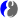# DScience:Introduction

Limitted access. First login to DataMelt if you are a full DataMelt member. Then login to HandWiki as a user.

40% complete

Descriptive statistics provides simple summaries about data. It is not used to derive complex characteristics Typically, descriptive statistics is used to measure central tendency and measures of spread around central values. Measures of central tendency include the mean, median/ Measures of variability include the standard deviation, variance, the minimum and maximum variables, and the kurtosis and skewness.

### Mean (average) value

The population mean, or expected value, is a measure of the central tendency either of a probability distribution or of the random variable characterized by that distribution. The arithmetic mean is defined as being equal to the sum of the numerical values of each and every observation divided by the total number of observations. Symbolically, if we have a data set containing the values $\displaystyle{ a_1, a_2, \ldots, a_n }$, then the arithmetic mean $\displaystyle{ A }$ is defined by the formula:

$\displaystyle{ A=\frac{1}{n}\sum_{i=1}^n a_i=\frac{a_1+a_2+\cdots+a_n}{n} }$

The arithmetic mean of a variable <amth>x[/itex] is often denoted by a bar, for example as in $\displaystyle{ \bar{x} }$ (read $\displaystyle{ x }$ bar). See further discussion ofArithmetic mean.

### Standard deviation

TheStandard deviation (also represented by the Greek letter $\displaystyle{ \sigma }$ or the Latin letter "s") is a measure used to quantify the amount of variation or dispersion of a set of data values. The formula for the sample standard deviation is

$\displaystyle{ s = \sqrt{\frac{\sum_{i=1}^N (x_i - \overline{x})^2}{N-1} }. }$

where $\displaystyle{ \textstyle\{x_1,\,x_2,\,\ldots,\,x_N\} }$ are the observed values of the sample items, $\displaystyle{ \textstyle\overline{x} }$ is the mean value of these observations, and N is the number of observations in the sample. A large standard deviation indicates that the data points can spread far from the mean and a small standard deviation indicates that they are clustered closely around the mean. The mean and the standard deviation of a set of data are usually reported together. The standard deviation is a "natural" measure of statistical dispersion if the center of the data is measured about the mean.

### KurtosisKurtosis (from Greek: κυρτός, kyrtos or kurtos, meaning "curved") is a measure of the "tailedness" of theProbability distribution of a real-valued random variable. The kurtosis is the fourthstandardized moment, defined as

$\displaystyle{ \operatorname{Kurt}[X] = \operatorname{E}\left[\left(\frac{X - \mu}{\sigma}\right)^4\right] = \frac{\mu_4}{\sigma^4} = \frac{\operatorname{E}[(X-\mu)^4]}{(\operatorname{E}[(X-\mu)^2])^2}, }$

where μ4 is the fourthcentral moment and σ is thestandard deviation. The kurtosis of anormal distribution is 3, therefore, it is useful to compare the kurtosis of a distribution to this value. Distributions with kurtosis less than 3 are said to be platykurtic.

This tutorial is provided under this license agreement.